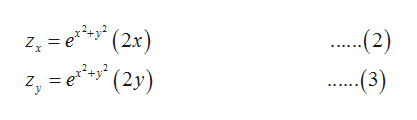Find an equation of the tangent plane to the given surface at the specified point.z=e^(x^2 + y^2) (1, −1, 1)

Question

Find an equation of the tangent plane to the given surface at the specified point.

z=e^(x^2 + y^2)

 (1, −1, 1)
Step 1

Given equation of the surface is,

Step 2

And the given points is (1,-1,1).

Partially differentiate the equation of the surface with respect to x and y,help_outlineImage Transcriptionclose= e(2x) '(2y) (2) Zx .(3) Z fullscreen
Step 3

Equation of the tangent plan...

Want to see the full answer?

See Solution

Want to see this answer and more?

Our solutions are written by experts, many with advanced degrees, and available 24/7

See Solution
Tagged in

Math